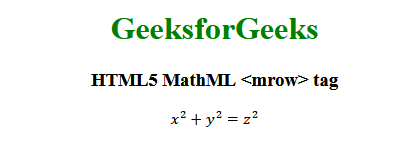Skip to content
Related Articles
HTML5 | MathML <mrow> Tag
• Last Updated : 07 Nov, 2019

The MathML <mrow> tag in HTML5 is used to create a row that contains some mathematical expression or any random text. When you need to write some mathematical expressions, this tag is useful to make a grouping of the expression. By using <mrow> the expression will look nicer, clean with indentation plus line breaking.

Syntax:

`<mrow> child elements </mrow>`

Attributes: This tag accepts some attributes which are listed below:

• class|id|style: This attribute is used to hold the styles of the child elements.
• href: This attribute is used to hold any hyperlink to a specified URL.
• mathbackground: This attribute holds the value of the math expressions background color.
• mathcolor: This attribute holds the color of the math expressions.
• dir: This attributes holds the direction value. It holds two types of direction values ltr for left to right and rtl for right to left.

Below example will illustrates the concept of HTML5 MathML <mrow> tag:

Example:

 ```<``html``>`` ` `<``head``>``    ``<``title``>HTML5 MathML mrow tag```` ` `<``body` `style``=``"text-align:center;"``>`` ` `    ``<``h1` `style``=``"color:green"``>GeeksforGeeks``     ` `    ``<``h3``>HTML5 MathML <``mrow``> tag``     ` `    ``<``math``>``        ``<``mrow``>``            ``<``mrow``>``                ``<``msup``>``                    ``<``mi``>x``                    ``<``mn``>2``                ````                ``<``mo``>+``                ``<``msup``>``                    ``<``mi``>y``                    ``<``mn``>2``                ````            ````            ``<``mo``>=``            ``<``msup``>``                ``<``mi``>z``                ``<``mn``>2``            ````        ````    `````` ` ``

Output:Supported Browsers: The browsers supported by HTML5 MathML <mrow> tag are listed below:

• Firefox

My Personal Notes arrow_drop_up